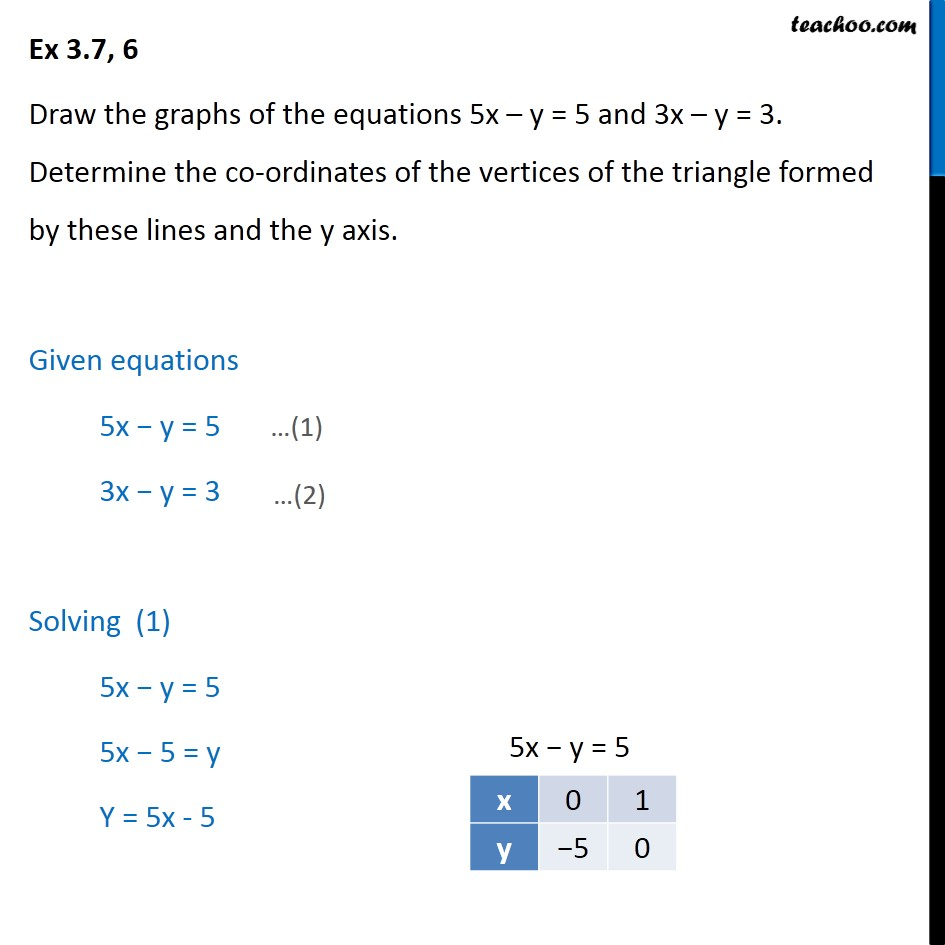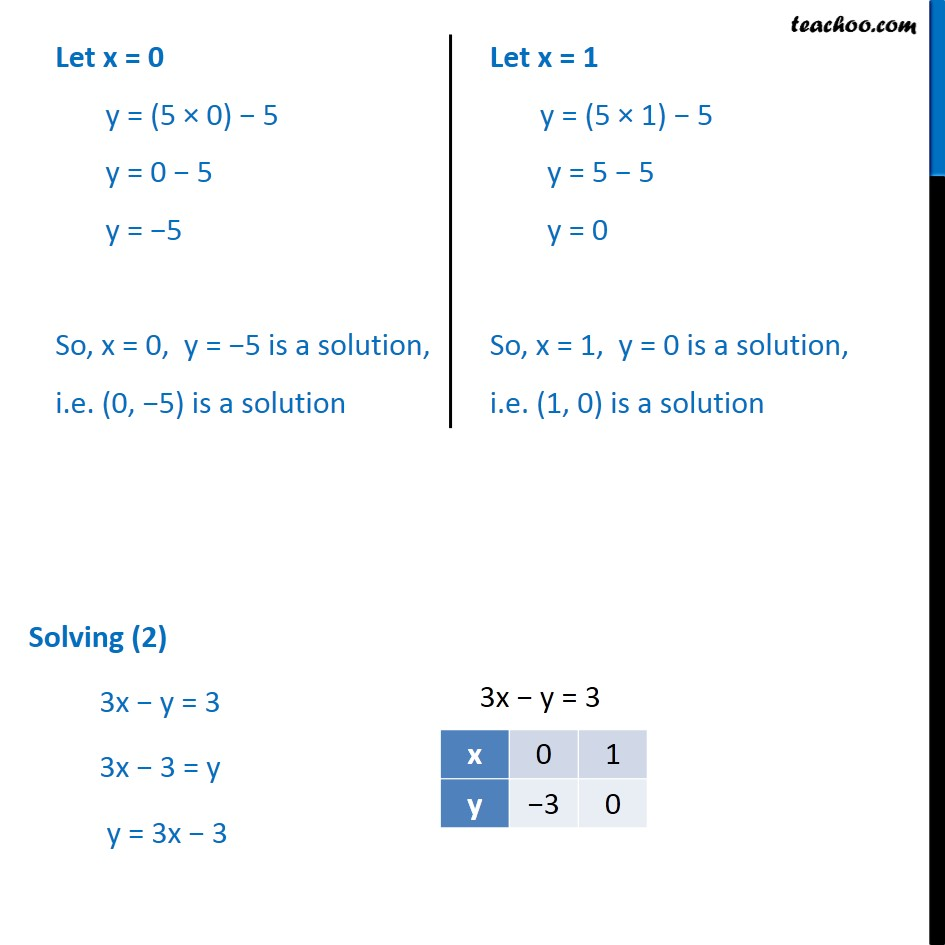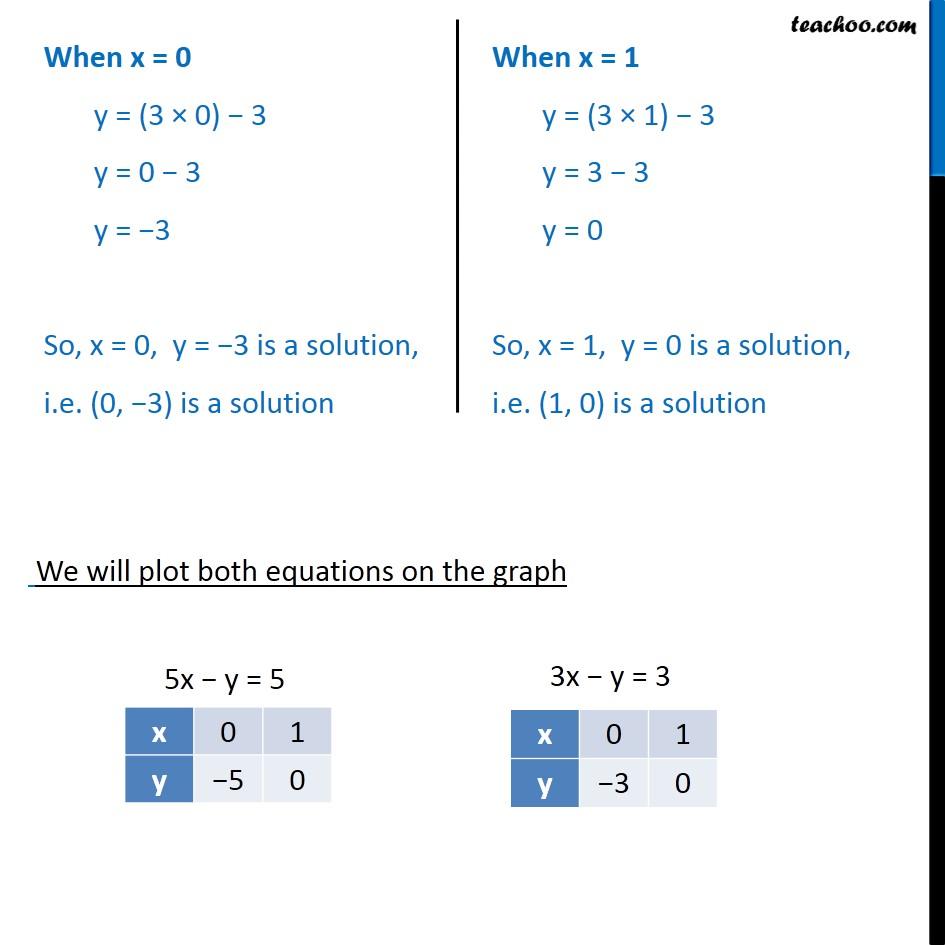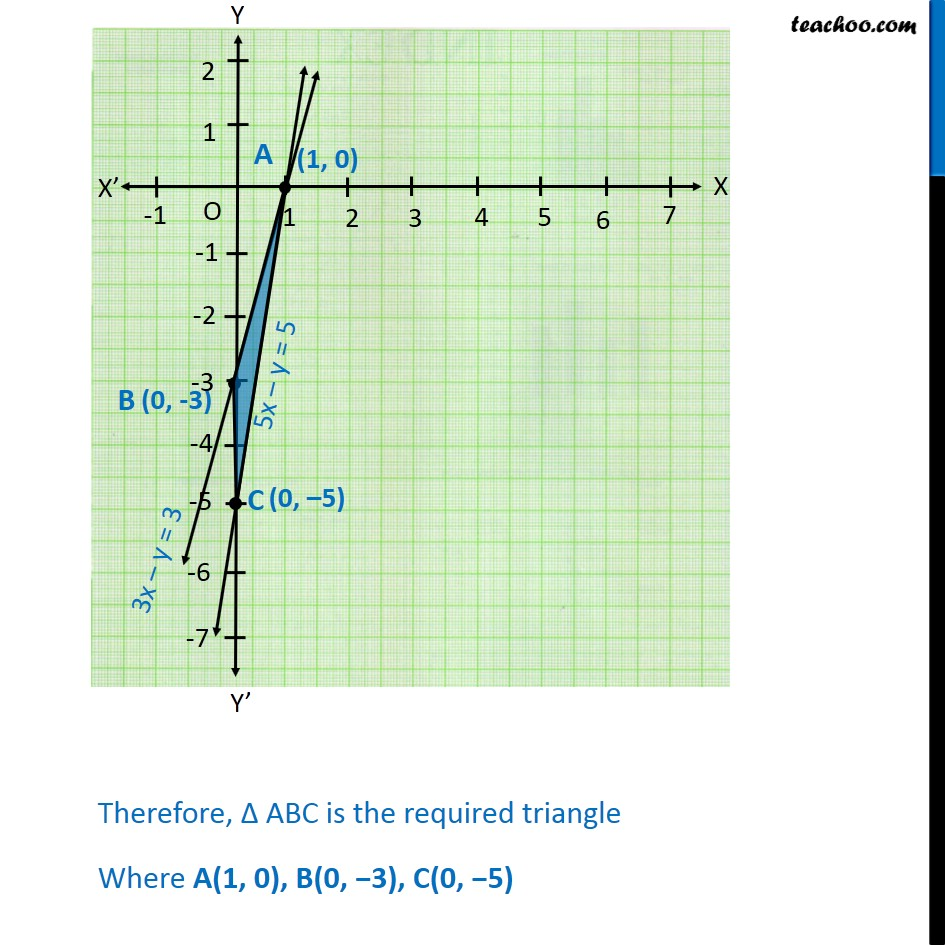1. Chapter 3 Class 10 Pair of Linear Equations in Two Variables
2. Serial order wise
3. Ex.3.7 (Optional)

Transcript

Ex 3.7, 6 Draw the graphs of the equations 5x y = 5 and 3x y = 3. Determine the co-ordinates of the vertices of the triangle formed by these lines and the y axis. Given equations 5x y = 5 3x y = 3 Solving (1) 5x y = 5 5x 5 = y Y = 5x - 5 Let x = 0 y = (5 0) 5 y = 0 5 y = 5 So, x = 0, y = 5 is a solution, i.e. (0, 5) is a solution Let x = 1 y = (5 1) 5 y = 5 5 y = 0 So, x = 1, y = 0 is a solution, i.e. (1, 0) is a solution Solving (2) 3x y = 3 3x 3 = y y = 3x 3 When x = 0 y = (3 0) 3 y = 0 3 y = 3 So, x = 0, y = 3 is a solution, i.e. (0, 3) is a solution When x = 1 y = (3 1) 3 y = 3 3 y = 0 So, x = 1, y = 0 is a solution, i.e. (1, 0) is a solution Therefore, ABC is the required triangle Where A(1, 0), B(0, 3), C(0, 5)

Ex.3.7 (Optional)

About the AuthorDavneet Singh
Davneet Singh is a graduate from Indian Institute of Technology, Kanpur. He has been teaching from the past 9 years. He provides courses for Maths and Science at Teachoo.